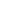SBI Annuity Deposit Calculator - Calculate your Annuity online in India | iNVESTT.in

# SBI Annuity Deposit Calculator

Rs.

%

Ys.

## What is SBI Annuity Deposit Scheme?

Anyone who wants to make use of the savings to provide income every month ought to go by registering an SBI annuity deposit scheme. The depositor will need to deposit a lumpsum and receive monthly income through the scheme.

After the deposit is made, a set amount will be divided into monthly installments that will be paid by depositor. This will mainly include the amount of interest earned along with the principal amount. Thus, you will get the deposits and the return of interest as monthly installments.

## Fixed Deposit Vs SBI Annuity Deposit Scheme:

In Fixed Deposit when we deposit some money in bank, in that case bank will give our deposited amount after the maturity date is completed but in SBI Annuity Deposit Scheme, when we deposit money in bank. Bank will give partial principle amount along with the interest earned every month as a income.

Partial Principle Amount + Interest Amount = Monthly Income

In SBI Annuity Deposit Scheme there’s no benefit once the maturity date is completed.

Let’s understand this with the help of an example-

You have deposited of Rs. 10, 00000/- with the maturity period of 7 years. The expected rate of interest is 7%. In that case the monthly pension will be Rs. 15092.

# CalculatorsSIP CalculatorLumpsum CalculatorStep SIP CalculatorTarget SIP CalculatorPPF CalculatorSukanya Samriddhi Yojana CalculatorHome Loan CalculatorCar Loan CalculatorIRR CalculatorSBI Annuity Deposit Calculator